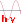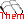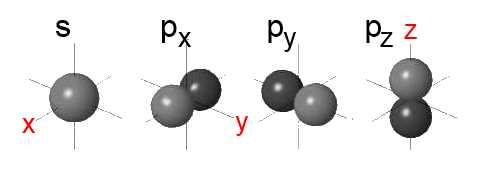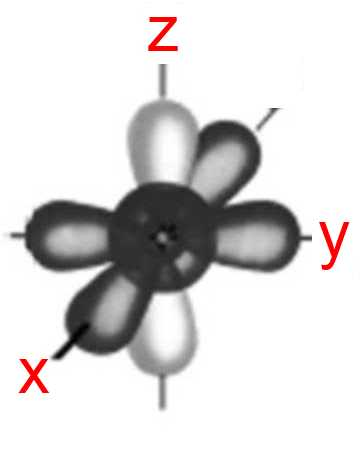Atomic orbitals

The term orbital refers to an energy state but also to a specific region in an atom where there is much chance of finding the electrons that occupy this orbital energy state.

Each orbital contains up to two electrons. The shape of the orbital has been calculated: - Orbitals $s$ are all spherically symmetric around the nucleus. Their size increases with the principal quantum number. - Orbitals $p_x$, $p_y$ and $p_z$ are oriented along the $x$, $y$ or $z$ axis and a have a form of a "dumbbell".- The other orbitals have more complicated shapes. In atoms, these orbitals interpenetrate, as for example in the case of the fluorine atom: (F $1s^2\;2s^2\;2p_x^2\;2p_y^2\;2p_z^1$):Orbitals $1s,\;2s,\;2p_x,\;2p_y$ with two electrons are here represented in dark gray, the orbital $2p_z$ with 1 electron in light gray. Clearly, the three $p$ orbitals are interchangeable.